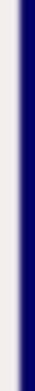homework help forum today's math problem links utilities previous math problem feedback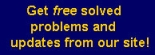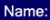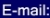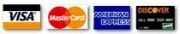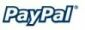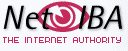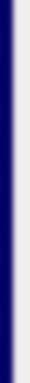# MATH HOMEWORK HELP

5. The price of a commodity is p dollars and the quantity supplied is x units, where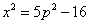. Find the rate of change of the quantity supplied with respect to time t (measured in months) when the price is \$4 and is increasing at the rate of 80 cents per month.

Solution: Using the chain rule we find that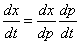From the given equation, and using the Chain Rule again, we find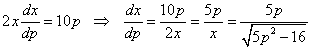Hence,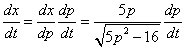But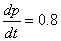and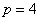, which means that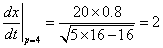MGT - Your source of Math and Statistics Homework Help